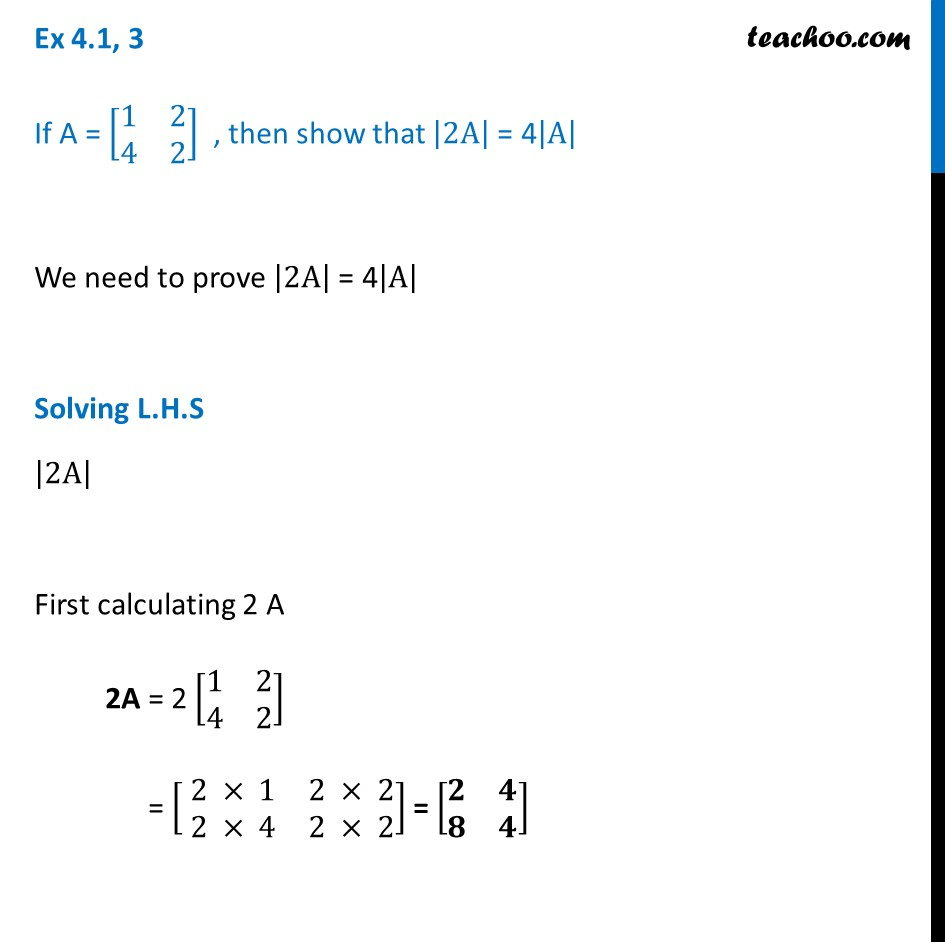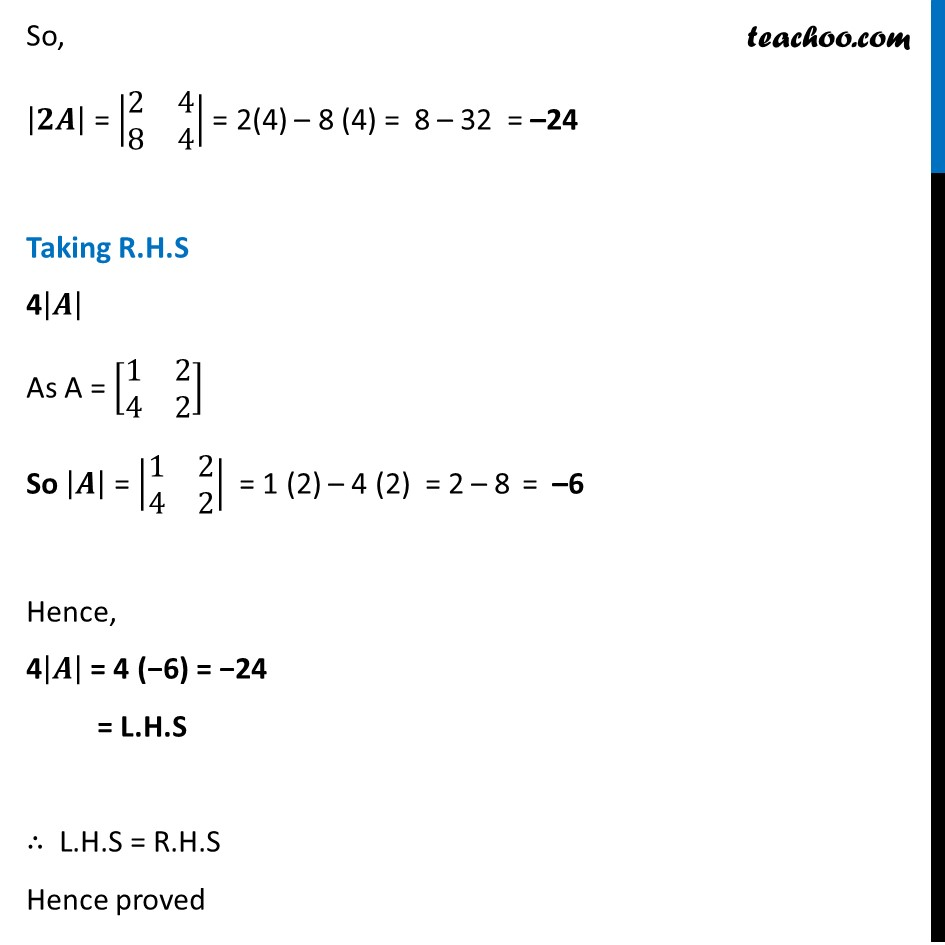Ex 4.1

Chapter 4 Class 12 Determinants
Serial order wiseLearn in your speed, with individual attention - Teachoo Maths 1-on-1 Class

### Transcript

Ex 4.1, 3 If A = [■8(1&2@4&2)] , then show that |2A| = 4|A| We need to prove |2A| = 4|A| Solving L.H.S |2A| First calculating 2 A 2A = 2 [■8(1&2@4&2)] = [■8( 2 × 1&2 × 2@ 2 × 4&2 × 2)] = [■8(𝟐&𝟒@𝟖&𝟒)] So, |𝟐𝑨| = |■8(2&4@8&4)| = 2(4) – 8 (4) = 8 – 32 = –24 Taking R.H.S 4|𝑨| As A = [■8(1&2@4&2)] So |𝑨| = |■8(1&2@4&2)| = 1 (2) – 4 (2) = 2 – 8 = –6 Hence, 4|𝑨| = 4 (−6) = −24 = L.H.S ∴ L.H.S = R.H.S Hence proved